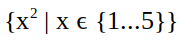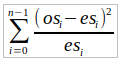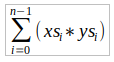# 列表理解 List Comprehensions

## Note:​

import Data.Char

## 基本概念​

There is also a video on this section.> [x^2 | x <- [1..5]][1,4,9,16,25]

Comprehension 可以有多个生成器，用逗号隔开。比如说：

> [(x,y) | x <- [1,2,3], y <- [4,5]][(1,4),(1,5),(2,4),(2,5),(3,4),(3,5)]

> [(x,y) | y <- [4,5], x <- [1,2,3]][(1,4),(2,4),(3,4),(1,5),(2,5),(3,5)]

## 依赖性生成器 Dependent Generators​

There is also a video on this section.

> [(x,y) | x <- [1..3], y <- [x..3]][(1,1),(1,2),(1,3),(2,2),(2,3),(3,3)]

concat :: [[a]] -> [a]concat xss = [x | xs <- xss, x <- xs]

For example:

> concat [[1,2,3],[4,5],][1,2,3,4,5,6]

firsts :: [(a,b)] -> [a]firsts ps = [x | (x, _) <- ps]

length :: [a] -> Intlength xs = sum [1 | _ <- xs]

## 卫士 Guards​

There is also a video on this section.

For example:

> [x | x <- [1..10], even x][2,4,6,8,10]

factors :: Int -> [Int]factors n = [x | x <- [1..n], n mod x == 0]

For example:

> factors 15[1,3,5,15]

prime :: Int -> Boolprime n = factors n == [1,n]

For example:

> prime 15False> prime 7True

primes :: Int -> [Int]primes n = [x | x <- [2..n], prime x]

For example:

> primes 40[2,3,5,7,11,13,17,19,23,29,31,37]

## 压缩函数 The Zip Function​

There is also a video on this section.

zip :: [a] -> [b] -> [(a,b)]

For example:

> zip ['a','b','c'] [1,2,3,4][('a',1),('b',2),('c',3)]

pairs :: [a] -> [(a,a)]pairs xs = zip xs (tail xs)

For example:

> pairs [1,2,3,4][(1,2),(2,3),(3,4)]

sorted :: Ord a => [a] -> Boolsorted xs = and [x <= y | (x,y) <- pairs xs]

For example:

> sorted [1,2,3,4]True> sorted [1,3,2,4]False

positions :: Eq a => a -> [a] -> [Int]positions x xs =   [i | (x',i) <- zip xs [0..], x == x']

For example:

> positions 0 [1,0,0,1,0,1,1,0][1,2,4,7]> positions False [True, False, True, False][1,3]

## 字符串的理解 String Comprehensions​

There is also a video on this section.

> "abcde" !! 2'c'> take 3 "abcde""abc"> length "abcde"5> zip "abc" [1,2,3,4][('a',1),('b',2),('c',3)]

count :: Char -> String -> Intcount x xs = length [x' | x' <- xs, x == x']

For example:

> count 's' "Mississippi"4

lowers :: String -> Intlowers xs = length [x | x <- xs, x >= 'a' && x <= 'z']

For example

> lowers "Haskell"6

## 扩展编程实例--凯撒密码 Extended Programming Example - The Caesar Cipher​

There is also a video on this section.

"haskell is fun" would be encoded as "kdvnhoo lv ixq" (for n = 3).

"haskell is fun" would be encoded as "rkcuovv sc pex" (for n = 10).

### 编码和解码 Encoding and Decoding​

import Data.Char

let2int :: Char -> Intlet2int c = ord c - ord 'a'int2let :: Int -> Charint2let n = chr (ord 'a' + n)

For example

> let2int 'a'0> int2let 0'a'

shift :: Int -> Char -> Charshift n c | isLower c = int2let ((let2int c + n) mod 26)          | otherwise = c

For example:

> shift 3 'a''d'> shift 3 'z''c'> shift (-3) 'c''z'

encode :: Int -> String -> Stringencode n xs = [shift n x | x <- xs]

For example:

> encode 3 "haskell is fun""kdvnhoo lv ixq"

For example:

> encode (-3) "kdvnhoo lv ixq""haskell is fun"

### 频率表 Frequency Tables​

There is also a video on this section.

table :: [Float]table = [8.1, 1.5, 2.8, 4.2, 12.7, 2.2, 2.0, 6.1, 7.0,         0.2, 0.8, 4.0, 2.4, 6.7, 7.5, 1.9, 0.1, 6.0,         6.3, 9.0, 2.8, 1.0, 2.4, 0.2, 2.0, 0.1]

percent :: Int -> Int -> Floatpercent n m = (fromIntegral n / fromIntegral m) * 100

For example:

> percent 5 1533.333336

freqs :: String -> [Float]freqs xs = [percent (count x xs) n | x <- ['a'..'z']]           where n = lowers xs

For example:

> freqs "abbcccddddeeeee"[6.666667, 13.333334, 20.0, 26.666667, ..., 0.0]

### 破解密码 Cracking the Cipher​

There is also a video on this section.chisqr :: [Float] -> [Float] -> Floatchisqr os es = sum [((o-e)^2)/e | (o,e) <- zip os es]

rotate :: Int -> [a] -> [a]rotate n xs = drop n xs ++ take n xs

For example:

> rotate 3 [1,2,3,4,5][4,5,1,2,3]

For example, if we let

table' = freqs "kdvnhoo lv ixq"

then

[chisqr (rotate n table') table | n <- [0..25]]

gives the result

[1408.8524, 640.0218, 612.3969, 202.42024, ..., 626.4024]

crack :: String -> Stringcrack xs = encode (-factor) xs  where     factor = head (positions (minimum chitab) chitab)     chitab = [chisqr (rotate n table') table | n <- [0..25]]     table' = freqs xs

For example:

> crack "kdvnhoo lv ixq""haskell is fun"> crack "vscd mywzboroxcsyxc kbo ecopev""list comprehensions are useful"

For example:

> crack (encode 3 "haskell")"piasmtt"> crack (encode 3 "boxing wizards jump quickly")"wjsdib rduvmyn ephk lpdxfgt"

## 练习​

(1) 如果 $x^2 + y^2 = z^2$，那么正整数的三重（x,y,z）被称为 pythagorean。使用列表理解法，定义一个函数：

pyths :: Int -> [(Int,Int,Int)]

For example:

> pyths 5[(3,4,5),(4,3,5)]

pyths :: Int -> [(Int,Int,Int)]pyths n = [(x,y,z) | x <- ns, y <- ns, z <- ns, x^2 + y^2 = z^2] where ns <- [1 .. n]

(2) 如果一个正整数等于其所有因子的总和，不包括数字本身，那么它就是完美的。使用列表理解法，定义一个函数

perfects :: Int -> [Int]

> perfects 500[6,28,496]

divisors :: Int -> [Int]divisors n = [x | x <- [0 .. n], n mod x == 0]perfects :: Int -> [Int]perfects n = [x | x <- [1 .. n], sum (divisors x) == x]

(3) 两个长度为 n 的整数列表 xs 和 ys 的标量乘积由相应整数的乘积之和给出：f :: [Int] -> [Int] -> Intf n m = sum [i * j | (i, j) <- zip n m]